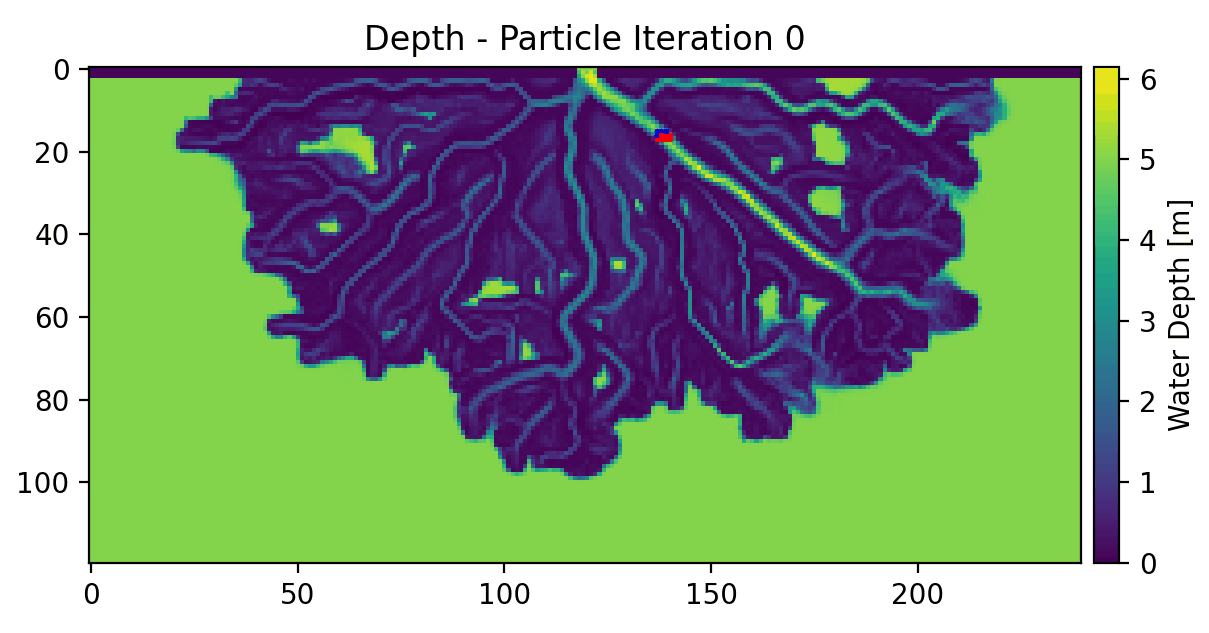# Example 2 - Particles in Steady Flow (DeltaRCM)¶

In this example, particles movement is simulated on a DeltaRCM model output. In this case, we have a developed river delta, and are going to be seeding particles in one of the main channels.

Full example script available `here`.

First the variables from the DeltaRCM model must be loaded. The example data for this case is provided in the “examples/” directory of the repository.

```>>> import numpy as np
>>> stage = data['stage']
>>> depth = data['depth']
```

Now we will create the parameter class and assign attributes to it.

```>>> params = pt.modelParams()
>>> params.stage = stage
>>> params.depth = depth
>>> params.dx = 50.
>>> params.model = 'DeltaRCM'
```

In this example case we haven’t packaged any flow data, so we are going to see how the particles can be routed just on the basis of water depth and water surface slope.

```>>> params.qx = np.zeros(np.shape(params.depth))
>>> params.qy = np.zeros(np.shape(params.depth))
```

Now that the parameters have all been defined, we will define the particles class and generate a set of particles.

```>>> seed_xloc = list(range(15, 17))
>>> seed_yloc = list(range(137, 140))
>>> Np_tracer = 50
>>> particles = pt.Particles(params)
>>> particles.generate_particles(Np_tracer, seed_xloc, seed_yloc)
```

we will route the particles for 50 iterations. An animation of this output is provided below.

```>>> walk_data = steady_plots(particles, 50, 'steady_deltarcm_example')
```